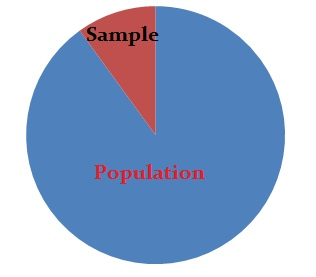# 10% Condition in Statistics: What is it?

Statistics Definitions > 10% Condition

## 10% Condition

The 10% condition states that sample sizes should be no more than 10% of the population. Whenever samples are involved in statistics, check the condition to ensure you have sound results. Some statisticians argue that a 5% condition is better than 10% if you want to use a standard normal model.For example, the 10% condition normally applies when you:

The 10% condition isn’t normally checked for:

• Chi-square tests
• Differences of means (except for small populations or for extremely large samples).
• Randomized experiments (there is no sampling in randomized experiments, so the 10% condition can’t be used).

Usually, you won’t find the 10% condition mentioned for statistical means. When you make inferences about proportions, the 10% condition is necessary because of the large samples. But for means, the samples are usually smaller, making the condition necessary only if you are sampling from a very small population.

The condition applies in Bernoulli trials because in the vast majority of cases you sample without replacement, For example, in a telephone survey asking “yes” or “no,” you don’t put a person who has already answered the question back in the pool.

### Where does the 10 percent condition come from?

The condition is statistically sound because of the mathematical proofs behind the assumption. The proof is a little beyond an elementary statistics or AP statistics class, but if you are really interested in knowing the mechanics behind the condition, The University of Texas has a pretty good rundown on the math.

## References

Kotz, S.; et al., eds. (2006), Encyclopedia of Statistical Sciences, Wiley.
Lindstrom, D. (2010). Schaum’s Easy Outline of Statistics, Second Edition (Schaum’s Easy Outlines) 2nd Edition. McGraw-Hill Education

CITE THIS AS:
Stephanie Glen. "10% Condition in Statistics: What is it?" From StatisticsHowTo.com: Elementary Statistics for the rest of us! https://www.statisticshowto.com/10-condition/